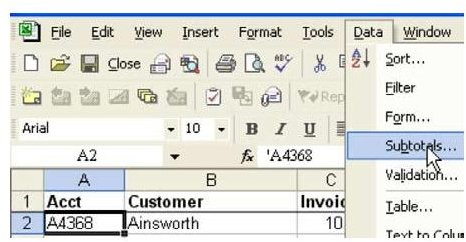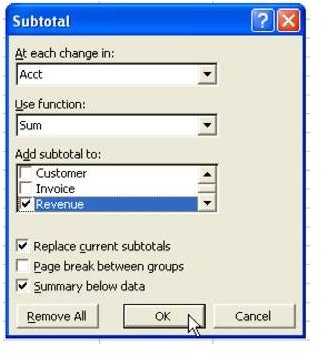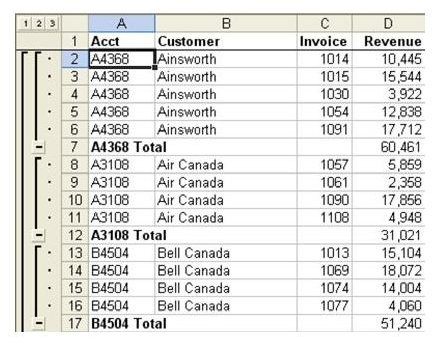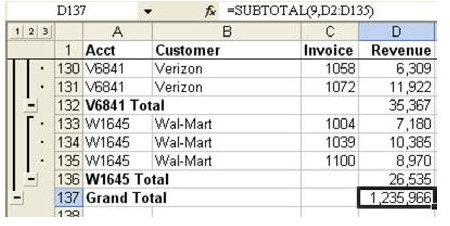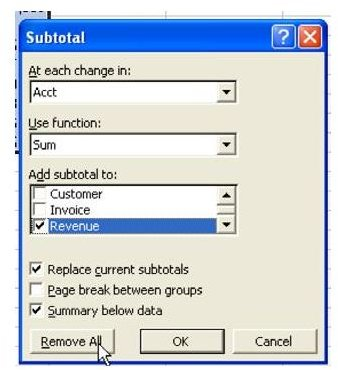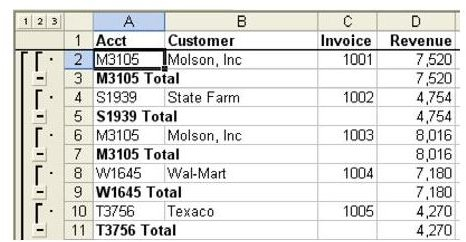# Discover How To Add Subtotals To A Dataset With This Excel Tutorial

Page content

See all Microsoft Excel tips

Strategy: A gift from Microsoft in Excel 95, the Automatic Subtotals feature will solve this problem in seconds instead of minutes.

By design, the dataset shown above is optimized for using the Subtotals command. Be sure to read the remaining examples for more details.

1. Start with data in list format. Select a single cell in the dataset. From the menu, select Data – Subtotals, as shown in Fig. 665.

2. The Subtotals dialog assumes that you want to subtotal by the field in the leftmost column of your data. It also assumes that you want to total the rightmost field. In the present example, shown in Fig. 666, this is correct, so you can choose OK.

In 1-2 seconds, subtotals will be inserted at each change in customer, as shown in Fig. 667.

If you scroll to the end of the dataset, you will notice that Excel added a grand total of all customers, as shown in Fig. 668.

As shown in Fig. 668, the inserted rows are using the somewhat new SUBTOTAL function. This function will total all of the cells in the range except for cells that contain other SUBTOTAL functions.

Additional Details:In order to remove subtotals, select a cell in the dataset. From the menu, select Data – Subtotals. In the Subtotals dialog, choose the Remove All button, as shown in Fig. 669.

Gotcha: This example worked because the data was sorted by Account Number. If the data had been sorted by Invoice Number instead, the result would have been fairly meaningless, as shown in Fig. 670.

Summary: The Subtotals command on the data menu quickly automates the monotonous job of adding subtotals.

Commands Discussed: Data – Subtotals

Functions Discussed: =Subtotal()

See all Microsoft Excel tips

## Images